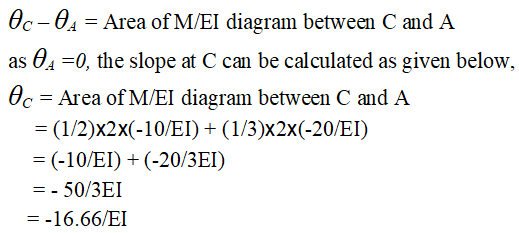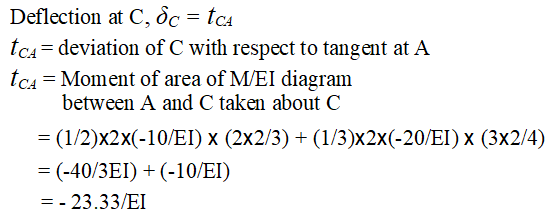# Slope and Deflection by Moment Area Theorems

Problem 7-6: Use moment-area theorems to determine the slope and deflection at point C of the cantilever beam given above in Figure 7-6(a)Solution:

Step 1: The M/EI (bending moment divided by EI) diagram is shown in figures 7-6(b) due to point load and 7-6(c) due to uniformly distributed load (udl). We will use principle of superposition to find resultant M/EI. The elastic curve (deflected shape of beam) is shown in figure 7-6(d).  As the point A is fixed it will have zero slope hence the tangent to the elastic curve will be horizontal at A as shown in the elastic curve in figure 7-6(d).Step 2: According to first moment-area theorem, the change in slope of two points on the elastic curve is equal to the area of M/EI diagram between these two points.

Hence change in slope between A and C is equal to the area of M/EI diagram between A and C. The M/EI diagram due to point load shown in Figure 7-6(b) is a triangle (area =bh/2) whereas the M/EI diagram due to udl shown in Figure 7-6(c) is parabolic (area=bh/3) . Hence we can write as follows,It is to be noted that the negative sign of M/EI is used because the bending moment is hogging due to point load and uniform load. The negative sign of slope indicates that it is clock-wise.

Step 3: The deflection at C can be calculated by using second moment area theorem which says that tangential deviation of a point C with respect to tangent at A is equal to the moment of area of M/EI diagram between A and C taken about C.

READ  Calculator for Deflection and slope of Simply Supported Beam

It is clear from the elastic curve in Figure 7-6(d) that the deflection at point C is equal the tangential deviation of point C with respect to tangent at A .

Hence deflection at C is calculated as given below.The negative sign of deviation indicates that it is below the tangent. Hence the deflection is in the downward direction.

You can also solve this problem by using online calculator for slope and deflection.